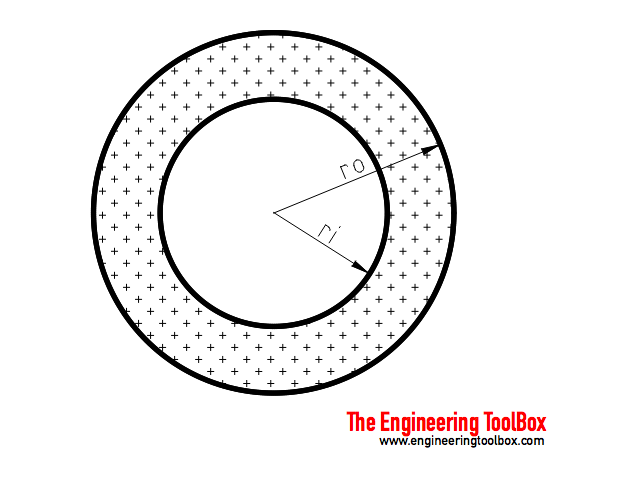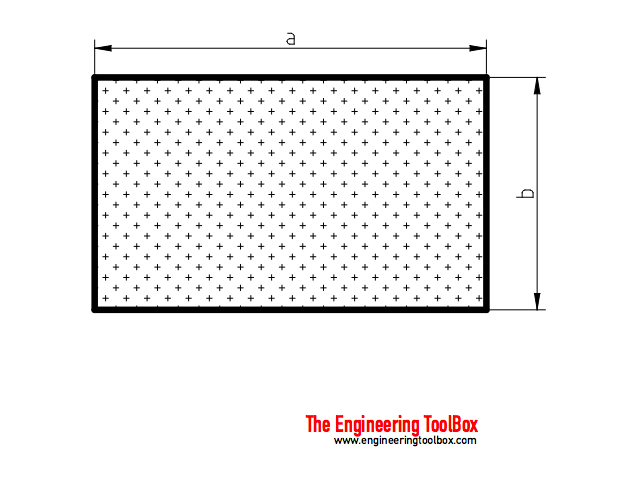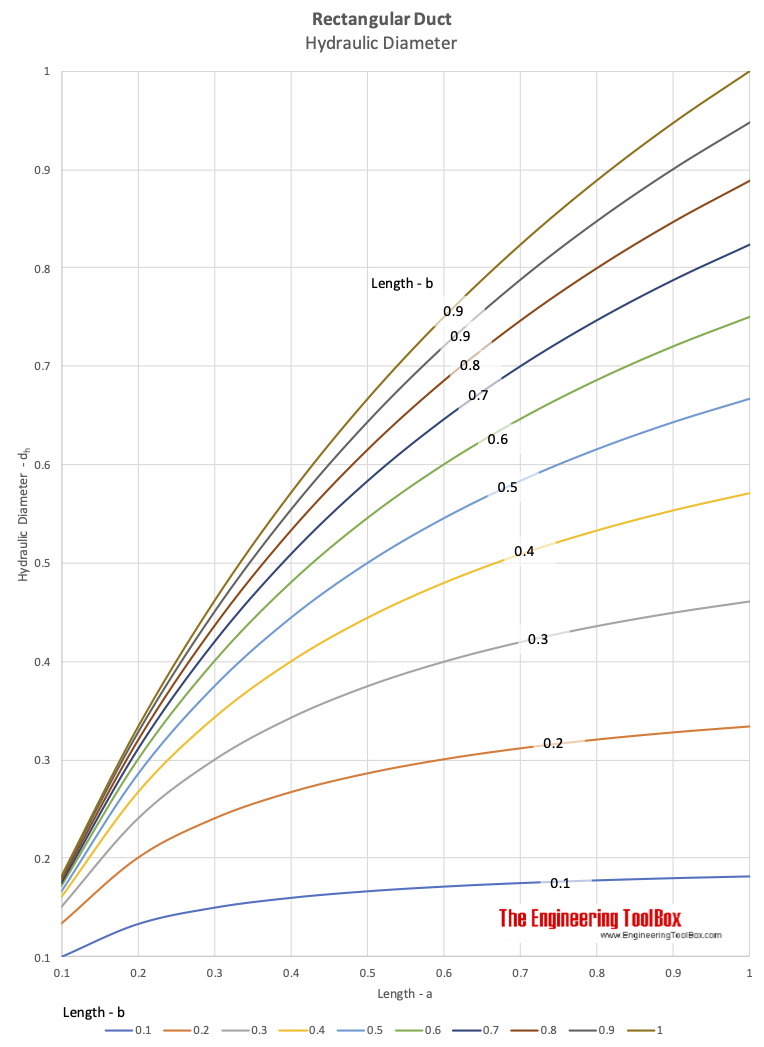Engineering ToolBox - Resources, Tools and Basic Information for Engineering and Design of Technical Applications!

# Fluid Flow - Hydraulic Diameter

## Calculate hydraulic diameter for pipes and ducts.

Hydraulic diameter - dh - is the "characteristic length" used to calculate the dimensionless Reynolds Number to determine if a flow is turbulent or laminar. A flow is

• laminar if Re < 2300
• transient for 2300 < Re < 4000
• turbulent if Re > 4000

Note that the velocity in the Reynolds equation is based on actual cross section area of duct or pipe.

The hydraulic diameter is used the Darcy-Weisbach equation to calculate pressure loss in ducts or pipes.

Note! - the hydraulic diameter is not the same as the geometrical equivalent diameter of non-circular ducts or pipes.

Hydraulic diameter can be calculated with the generic equation

dh = 4 A / p                               (1)

where

dh = hydraulic diameter (m, ft)

A = area section of the duct or pipe (m2, ft2)

p = "wetted" perimeter of the duct or pipe (m, ft)

Note! - inches are commonly used in the Imperial unit system

### Hydraulic Diameter of a Circular Tube or DuctBased on equation (1) the hydraulic diameter of a circular duct can be expressed as:

dh = 4 π r2 / 2 π r

= 2 r

= d                                  (2)

where

r = pipe or duct inside radius  (m, ft)

d = pipe or duct inside diameter  (m, ft)

As we could expect the hydraulic diameter of a standard circular tube or duct is the inner diameter or two times the inner radius.

### Hydraulic Diameter of Circular Tube with Circular Tube on the Inside

The flow is in the volume between the inside and outside pipe.Based on equation (1) the hydraulic diameter of a circular duct or tube with an inside duct or tube can be expressed as

dh = 4 (π ro2 - π ri2) / (2 π ro + 2 π ri)

= 2 (ro - ri)                                        (3)

where

ro = inside radius of the outside tube (m, ft)

ri = outside radius of the inside tube (m, ft)

### Hydraulic Diameter of Rectangular Tubes or DuctsBased on equation (1) the hydraulic diameter of a rectangular duct or pipe can be calculated as

dh = 4 a b / (2 (a + b))

= 2 a b / (a + b)                                 (4)

where

a = width/height of the duct (m, ft)

b = height/width of the duct (m, ft)

### Related Mobile Apps from The EngineeringToolBox

- free apps for offline use on mobile devices.

#### Rectangular to Circulate Duct/Tube Hydraulic Diameter Calculator

The calculator below is based on formula (4) an can be used to calculate the hydraulic diameter of rectangular duct or tube. The formula is generic and any unit can be used.

Length side a - (m, ft)

Length side - b - (m, ft)### Equivalent diameter

Note! The hydraulic diameter is not the same as the equivalent diameter. The equivalent diameter is the diameter of a circular duct or pipe that gives the same pressure loss as a rectangular duct or pipe.

## Related Topics

• Fluid Mechanics - The study of fluids - liquids and gases. Involving velocity, pressure, density and temperature as functions of space and time.
• Fluid Flow and Pressure Loss - Pipe lines - fluid flow and pressure loss - water, sewer, steel pipes, pvc pipes, copper tubes and more.
• Ventilation - Systems for ventilation and air handling - air change rates, ducts and pressure drops, charts and diagrams and more.

## Engineering ToolBox - SketchUp Extension - Online 3D modeling!

Add standard and customized parametric components - like flange beams, lumbers, piping, stairs and more - to your Sketchup model with the Engineering ToolBox - SketchUp Extension - enabled for use with the amazing, fun and free SketchUp Make and SketchUp Pro .Add the Engineering ToolBox extension to your SketchUp from the SketchUp Pro Sketchup Extension Warehouse!

Translate

## Privacy

We don't collect information from our users. Only emails and answers are saved in our archive. Cookies are only used in the browser to improve user experience.

Some of our calculators and applications let you save application data to your local computer. These applications will - due to browser restrictions - send data between your browser and our server. We don't save this data.

## Citation

• Engineering ToolBox, (2003). Fluid Flow - Hydraulic Diameter. [online] Available at: https://www.engineeringtoolbox.com/hydraulic-equivalent-diameter-d_458.html [Accessed Day Mo. Year].

Modify access date.

. .

#### Scientific Online Calculator3 30